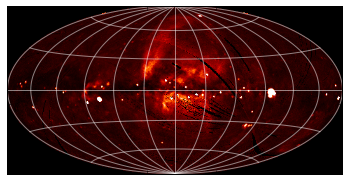# Astropy: WCS Transformations - Solutions¶

## Level 1¶

The final pixel coordinates should always agree with the starting ones, since each pixel covers a unique world coordinate position. The world coordinates of the pixel at (1, 1) are not defined:

In :
from astropy.wcs import WCS
w = WCS('data/ROSAT.fits')
w.wcs_pix2world(1, 1, 1)

Out:
[array(nan), array(nan)]

because the pixel lies outside the coordinate grid. Thus, not all pixels in an image have a valid position on the sky.

## Level 2¶

In :
import numpy as np
from astropy.io import fits
from astropy.wcs import WCS
from astropy.table import Table

# Read in LAT Point Source Catalog

# Extract Galactic Coordinates
l = psc['GLON']
b = psc['GLAT']

hdulist_im = fits.open('data/ROSAT.fits')

image = hdulist_im.data

# Instantiate WCS object

# Find pixel positions of LAT sources. Note we use 0 here for the last
# argument, since we want zero based indices (for Numpy), not the FITS
# pixel positions.
px, py = w.wcs_world2pix(l, b, 0)

# Find the nearest integer pixel
px = np.round(px).astype(int)
py = np.round(py).astype(int)

# Find the ROSAT values (note the reversed index order)
values = image[py, px]

# Print out the values
print(values)

WARNING: hdu= was not specified but multiple tables are present, reading in first available table (hdu=1) [astropy.io.fits.connect]

[ 123.7635498   163.27642822  221.76609802 ...,  255.07995605  100.35219574
87.62506104]

WARNING: UnitsWarning: 'photon/cm**2/MeV/s' contains multiple slashes, which is discouraged by the FITS standard [astropy.units.format.generic]
WARNING: UnitsWarning: 'photon/cm**2/s' contains multiple slashes, which is discouraged by the FITS standard [astropy.units.format.generic]
WARNING: UnitsWarning: The unit 'erg' has been deprecated in the FITS standard. Suggested: cm2 g s-2. [astropy.units.format.utils]
WARNING: UnitsWarning: 'erg/cm**2/s' contains multiple slashes, which is discouraged by the FITS standard [astropy.units.format.generic]


## Level 3¶

In :
import numpy as np
%matplotlib inline
from matplotlib import pyplot as plt
from astropy.io import fits
from astropy.wcs import WCS

hdulist = fits.open('data/ROSAT.fits')

image = hdulist.data

# Instantiate WCS object

# Plot the image
fig = plt.figure()
ax.imshow(image, cmap=plt.cm.gist_heat,
origin='lower', vmin=0, vmax=1000.)

# Loop over lines of longitude
for lon in np.linspace(-180., 180., 13):
grid_lon = np.repeat(lon, 100)
grid_lat = np.linspace(-90., 90., 100)
px, py = w.wcs_world2pix(grid_lon, grid_lat, 1)
ax.plot(px, py, color='white', alpha=0.5)

# Loop over lines of latitude
for lat in np.linspace(-60., 60., 5):
grid_lon = np.linspace(-180., 180., 100)
grid_lat = np.repeat(lat, 100)
px, py = w.wcs_world2pix(grid_lon, grid_lat, 1)
ax.plot(px, py, color='white', alpha=0.5)

ax.set_xlim(0, image.shape)
ax.set_ylim(0, image.shape)
ax.set_xticklabels('')
ax.set_yticklabels('')
plt.show()